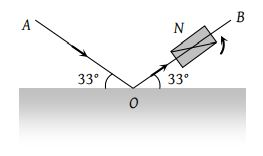What will be the angular width of central maxima in Fraunhoffer diffraction when light of wavelength $6000\text{\hspace{0.17em}}Å$ is used and slit width is 12×10–5 cm

Concept Questions :-

Diffraction
High Yielding Test Series + Question Bank - NEET 2020

Difficulty Level:

Direction of the first secondary maximum in the Fraunhofer diffraction pattern at a single slit is given by (a is the width of the slit)

(1) $a\mathrm{sin}\theta =\frac{\lambda }{2}$

(2) $a\mathrm{cos}\theta =\frac{3\lambda }{2}$

(3) $a\mathrm{sin}\theta =\lambda$

(4) $a\mathrm{sin}\theta =\frac{3\lambda }{2}$

Concept Questions :-

Diffraction
High Yielding Test Series + Question Bank - NEET 2020

Difficulty Level:

A parallel monochromatic beam of light is incident normally on a narrow slit. A diffraction pattern is formed on a screen placed perpendicular to the direction of the incident beam. At the first minimum of the diffraction pattern, the phase difference between the rays coming from the edges of the slit is

(1) $0$

(2) $\frac{\pi }{2}$

(3) $\pi$

(4) 2$\pi$

Concept Questions :-

Diffraction
High Yielding Test Series + Question Bank - NEET 2020

Difficulty Level:

A parallel beam of monochromatic light of wavelength 5000 Å is incident normally on a single narrow slit of width 0.001 mm. The light is focused by a convex lens on a screen placed on the focal plane. The first minimum will be formed for the angle of diffraction equal to

(1) 0o

(2) 15o

(3) 30o

(4) 60o

Concept Questions :-

Diffraction
High Yielding Test Series + Question Bank - NEET 2020

Difficulty Level:

In the far field diffraction pattern of a single slit under polychromatic illumination, the first minimum with the wavelength ${\lambda }_{1}$ is found to be coincident with the third maximum at ${\lambda }_{2}$. So

(1) $3{\lambda }_{1}=0.3{\lambda }_{2}$

(2) $3{\lambda }_{1}={\lambda }_{2}$

(3) ${\lambda }_{1}=3.5{\lambda }_{2}$

(4) $0.3{\lambda }_{1}=3{\lambda }_{2}$

Concept Questions :-

Diffraction
High Yielding Test Series + Question Bank - NEET 2020

Difficulty Level:

The angle of polarisation for any medium is 60o, what will be critical angle for this

(1) ${\mathrm{sin}}^{-1}\sqrt{3}$

(2) ${\mathrm{tan}}^{-1}\sqrt{3}$

(3) ${\mathrm{cos}}^{-1}\sqrt{3}$

(4) ${\mathrm{sin}}^{-1}\frac{1}{\sqrt{3}}$

Concept Questions :-

Polarization of light
High Yielding Test Series + Question Bank - NEET 2020

Difficulty Level:

A beam of light AO is incident on a glass slab (μ = 1.54) in a direction as shown in figure. The reflected ray OB is passed through a Nicol prism on viewing through a Nicole prism, we find on rotating the prism that(1) The intensity is reduced down to zero and remains zero

(2) The intensity reduces down some what and rises again

(3) There is no change in intensity

(4) The intensity gradually reduces to zero and then again increases

Concept Questions :-

Polarization of light
High Yielding Test Series + Question Bank - NEET 2020

Difficulty Level:

In the propagation of electromagnetic waves, the angle between the direction of propagation and plane of polarisation is

(1) 0o

(2) 45o

(3) 90o

(4) 180o

Concept Questions :-

Polarization of light
High Yielding Test Series + Question Bank - NEET 2020

Difficulty Level:

A light has amplitude A and the angle between analyzer and polariser is 60°. Light reflected by analyzer has amplitude

(1) $A\sqrt{2}$

(2) $A/\sqrt{2}$

(3) $\sqrt{3}A/2$

(4) A/2

Concept Questions :-

Polarization of light
High Yielding Test Series + Question Bank - NEET 2020

Difficulty Level:

Light passes successively through two polarimeters tubes each of length 0.29m. The first tube contains dextro rotatory solution of concentration 60kgm–3 and specific rotation 0.01rad m2kg–1. The second tube contains laevo rotatory solution of concentration 30kg/m3 and specific rotation 0.02 radm2kg–1. The net rotation produced is

(1) 15°

(2) 0°

(3) 20°

(4) 10°

Concept Questions :-

Polarization of light